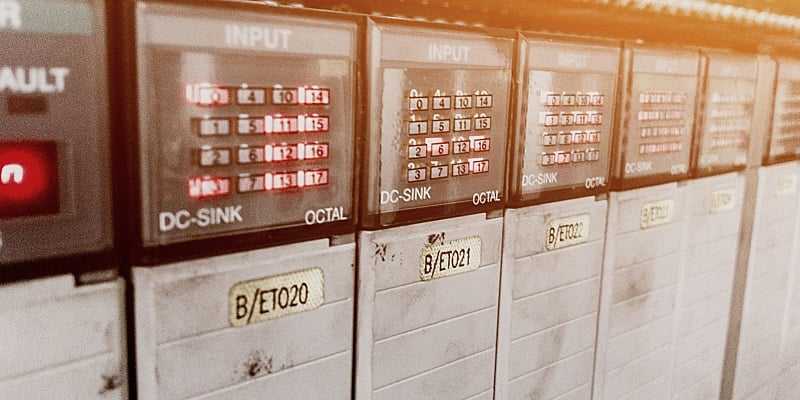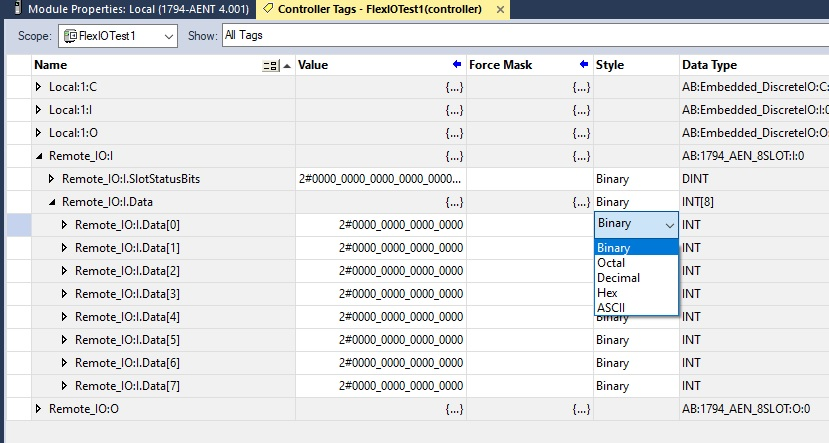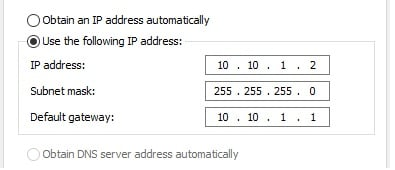Technical Article

# An Engineer Explains the Hexadecimal and Octal Number Systems

March 01, 2023 by David Peterson

## Computers understand values as long strings of binary bits, but we humans convert them to other systems for convenience. Decimal makes sense, but what about hexadecimal and the lesser-known octal systems?

The binary numbering system is typically known for its role in the world of IT. From programming to IP addresses, bits and bytes are a staple of the computer operating system.

But for control engineers, the questions about numbering systems are not answered in a typical computer textbook. Simply being able to calculate the decimal equivalent of a binary number using the ‘light switch’ analogy does not answer the fundamental questions of how it is used in automation.

Perhaps even more importantly: why?

### Bit and Byte Memory Storage

When computers were first designed, memory was stored as individual bits in vast arrays, hundreds or even thousands of addresses relating to each 1 and 0 of data.

Memory technology quickly advanced to the point where hundreds of thousands and millions of bits could be stored. To make more effective use of the memory storage and addressing functions, these bits were stored in groups of 8. Very few cases exist where less than 8 bits would be enough data to store a program. Well, except for us BOOL-loving engineers—but binary counting is the subject of other writings.##### Figure 1. Although less common for control engineering, it is still no surprise to encounter binary, hex, and even octal systems on the job. Image used courtesy of Canva

The groups of 8 bits were given a name: bytes. To this day, memory is referenced by kilobytes and megabytes and is always increasing.

These 8-bit bytes can store a value between 0000 0000 (equal to 0) and 1111 1111 (equal to 255). In some cases, they can represent a value, like a temperature or pressure from a sensor. They might also indicate a set of alarm statuses so that the decimal equivalent value would represent a unique combination of alarm codes. In other cases, an address designator, like an IP address.

### How Does the Hexadecimal Number System Work?

To simplify the numbering languages, displaying these bytes of information in fewer than eight digits was helpful. And for longer strings of bytes, even more space is conserved. It was chosen to break the values into two 4-bit groups. Four bits can equal a value between 0 and 15. For this reason, hex is called the ‘base 16’ number system.

Those familiar with the hexadecimal system recognize this 0 to 15, but it uses an alternative set of characters: 0 to F.

The problem with using values between 10-15 is that they are actually two numbers. The number 10 is really 1 and 0, each with a place value. To solve this problem, the numbers 10-15 are assigned letters A-F to ensure the computer and reader recognize them as one character.

In control system programming, hexadecimal values are very common to use in the representation of a device address and in the value of data being returned from a sensor or system. They will be displayed with a 0x, like 0x2F represents a byte—four bits for a 2 and four bits for an F.

Many industrial networks use byte addressing for devices; therefore, the maximum number of nodes is 255, with addresses from 0x00 to 0xFF.

It can be helpful to keep a chart of hexadecimal to decimal equivalents. Still, many PLC programs provide users with the option to represent data in either format, so a chart is unnecessary.##### Figure 2. Displaying control system data in one of several numbering formats.

For some data streams, a single byte is not enough. Consider temperature, where degrees may easily exceed 255 (C or F). So in these cases, it might be useful to store the data in a 2-byte integer, and these are commonly known as the 16-bit INT.

For these 16-bit values, the hex representation will be four characters long, such as 0x2F3C, for example.

#### Why Not Use Number Systems With 8 Bits?

Hex is one of the largest common numbering systems, in groups of 4 bits. Since the byte is so common, why not use such 8-bit groups?

The answer becomes clear when we consider that A-F was chosen to represent the values that extend beyond a single decimal digit. If we were to try and design a system with 255 different single-character digits, we would be forced to use all the upper and lowercase numbers, probably some Greek letters, which would be far more difficult than anyone would care to imagine.##### Figure 3. IP addresses, subnet masks, and other properties are represented as 8-bit groups, sometimes seen as two hexadecimal characters.

For that reason, we remain at base 16, the balance between consolidation and comprehension.

### What About the Octal Number System?

The octal, or ‘base 8’, numbering system is the lesser-used platform in control engineering. It uses only 3 bits in each group rather than 4. Therefore, instead of supporting values from 0-16 like hex, it is limited to a range of 0-7.

Since most computer values are multiple of 4, they fit the hex scheme very well, but far fewer that are limited to multiples of 3.

The reason for inclusion in some control architecture (although even then, only rarely seen) is the interoperability with legacy systems. When memory was more limited, integer (word) sizes were not always 8- and 16- bits as they are today. Some of them were indeed multiples of 3, such as 12-bit words, and octal could be useful for consolidating that data.

As to the use of octal in modern systems? I would be interested to learn about applications—please feel free to share them in the comment section.

### Understanding Number Systems

Certainly, some systems of numbers are more common than others. We can’t go a single day without using our familiar decimal system, and in the control engineering world, we frequently encounter binary as well.

Understanding the structure and application of hex values can also bring understanding to many product datasheets, EDS files, and communication protocols and paves the way for understanding other coding systems like ASCII, gray codes, and BCD, among others.